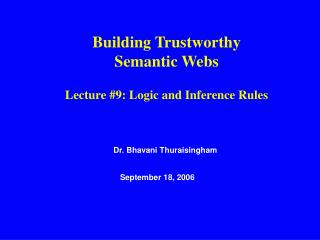DownloadDownload PresentationDr. Bhavani Thuraisingham

# Dr. Bhavani Thuraisingham

Download Presentation## Dr. Bhavani Thuraisingham

- - - - - - - - - - - - - - - - - - - - - - - - - - - E N D - - - - - - - - - - - - - - - - - - - - - - - - - - -
##### Presentation Transcript

1. Building Trustworthy Semantic Webs Lecture #9: Logic and Inference Rules Dr. Bhavani Thuraisingham September 18, 2006

2. Objective of the Unit • This unit will provide an overview of logic and inference rules component of the semantic web and then discuss some security issues

3. Outline of the Unit • What are logic and inference rules • Why do we need rules? • Example rules • Logic programs • Monotonic and Nonmonotoic rules • Rule Markup • Example Rule Markup in XML • Policy Specification • Relationship to the Inference and Privacy problems • Summary and Directions

4. Logic and Inference • First order predicate logic • High level language to express knowledge • Well understood semantics • Logical consequence - inference • Proof systems exist • Sound and complete • OWL is based on a subset of logic – descriptive logic

5. Why Rules? • RDF is built on XML and OWL is built on RDF • We can express subclass relationships in RDF; additional relationships can be expressed in OWL • However reasoning power is still limited in OWL • Therefore the need for rules and subsequently a markup language for rules so that machines can understand

6. Example Rules • Studies(X,Y), Lives(X,Z), Loc(Y,U), Loc(Z,U)  HomeStudent(X) • i.e. if John Studies at UTDallas and John is lives on Campbell Road and the location of Campbell Road and UTDallas are Richardson then John is a Home student • Note that Person (X)  Man(X) or Woman(X) is not a rule in predicate logic That is if X is a person then X is either a man of a woman. This can be expressed in OWL However we can have a rule of the form Person(X) and Not Man(X)  Woman(X)

7. Monotonic Rules •  Mother(X,Y) • Mother(X,Y)  Parent(X,Y) If Mary is the mother of John, then Mary is the parent of John Syntax: Facts and Rules Rule is of the form: B1, B2, ---- Bn  A That is, if B1, B2, ---Bn hold then A holds

8. Logic Programming • Deductive logic programming is in general based on deduction • i.e., Deduce data from existing data and rules • e.g., Father of a father is a grandfather, John is the father of Peter and Peter is the father of James and therefore John is the grandfather of James • Inductive logic programming deduces rules from the data • e.g., John is the father of Peter, Peter is the father of James, John is the grandfather of James, James is the father of Robert, Peter is the grandfather of Robert • From the above data, deduce that the father of a father is a grandfather • Popular in Europe and Japan

9. Nonmonotonic Rules • If we have X and NOT X, we do not treat them as inconsistent as in the case of monotonic reasoning. • For example, consider the example of an apartment that is acceptable to John. That is, in general John is prepared to rent an apartment unless the apartment ahs less than two bedrooms, is does not allow pets etc. This can be expressed as follows: •  Acceptable(X) • Bedroom(X,Y), Y<2  NOT Acceptable(X) • NOT Pets(X)  NOT Acceptable(X) • Note that there could be a contradiction. But with nonmotonic reasoning this is allowed.

10. Rule Markup • The various components of logic are expressed in the Rule Markup Language – RuleML • Both monotonic and nonmonotnic rules can be represented • Example representation of Fact P(a) - a is a parent <fact> <atom> <predicate>p</predicate> <term> <const>a</const> <term> <atom> </fact>

11. Policies in RuleML <fact> <atom> <predicate>p</predicate> <term> <const>a</const> <term> <atom> Level = L </fact>